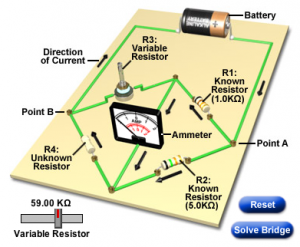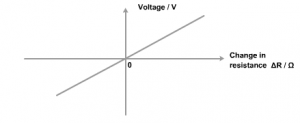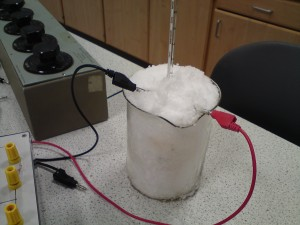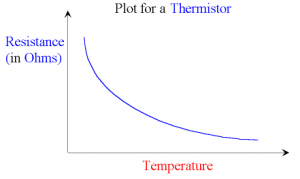# Higher – school closure work

With the school closure today, I’ve posted some revision work for the Wheatstone Bridge.  Please read through the revision section and attempt the questions in the attached pdf.

We’re coming to the end of the topic that investigates the behaviour of a Wheatstone Bridge. The bridge circuit is really just a pair of potential dividers (voltage dividers) dividers connected in parallel. A voltmeter, ammeter or galvanometer (very sensitive ammeter) connects the two potential divider chains together, as shown below.When the voltage (or current) displayed on the meter is zero, we say that the Wheatstone bridge is balanced. For a balanced bridge, it is possible to show that$\displaystyle {R_1 \over R_2} = \displaystyle {R_3 \over R_4}$

[you have this proof in p11 of your notes folder]

For the circuit shown above, the voltmeter will display the difference in electrical potential between points B and D. We can calculate this potential difference by finding the voltages at points B and D using the voltage divider equation you used for National5/Standard Grade/Intermediate 2 Physics.$V_2 = \displaystyle { R_2 \over {R_1+R_2}} \times V_s$

So in this example,$V_D = \displaystyle {R_2 \over {R_1+R_2}} \times V_s$

and$V_B = \displaystyle {R_X \over {R_3+R_X}} \times V_s$

The voltmeter displays the potential difference between these two points, i.e.$V_G = V_D-V_B$

Here is a short video that provides a recap of the Wheatstone Bridge.

and a worked example from an old SQA past paper

Now click on the picture below to try an interactive Wheatstone Bridge problem (you will need to have Java installed).Instructions:

• Press the Reset button to change the value of all the resistors in the circuit.
• Use the slider to balance the bridge. The circuit uses a centre-zero meter, so aim to get the indicator dead centre.
• Find the unknown resistance (R4) using the value of the other 3 resistors when the circuit is balanced.

You can repeat this simulation as many times as you like by pressing Reset to change the resistor values…..it’s great practice!

Here is an example of an application of the Wheatstone Bridge, called the metre bridge.

When a Wheatstone Bridge is slightly out of balance, it will provide a linear response. In other words, small changes in resistance will produce proportionally small changes in voltage or current. When these small changes are plotted, we obtain a straight line through the origin, like this:This property of a Wheatstone Bridge can be used to find the temperature of the physics classroom if one of the resistors is replaced with a thermistor – see p14 of your notes.  The thermistor is immersed in a beaker of ice & water (I used snow one year – see photo) and the Wheatstone Bridge is balanced while the thermistor is cold. Note the temperature of the ice/water mixture and the reading on the galvanometer.Without touching the resistance box settings, the thermistor is placed in warm water and the reading on the galvanometer is recorded, along with the water temperature.  Now plot these two points on a graph, with temperature on the x-axis and current on the y-axis.  Draw a straight line between the two points (I know, I know!)

Remove the thermistor from the beaker and leave it sitting on the desk for a few minutes.  Check the new galvanometer reading and use your graph to find which temperature would give this value for current.

When we did this on Monday (5.5 class), we found that the graph predicted a room temperature of 14°C, 20°C or 25°C, depending on the group.  The digital thermometer in the corner of the room showed 17°C.  Why is there a difference?

As we discussed on Monday, this experiment is flawed due to the way resistance of a thermistor changes with temperature (you might remember this from Nat5/SG/Int2).Since the resistance is not proportional to temperature, we will not get an accurate answer…..unless we stay very, very close to the temperature at which we balanced the Wheatstone Bridge. In this case, we tried to cover too large a range of temperatures.  For temperature ranges much smaller than the 80°C we attempted, it is possible to obtain an accurate estimate of room temperature.